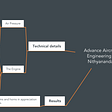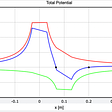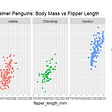# Swanson’s Swansong

Swanson’s rule is a commonly used device in a variety of risk and uncertainty management contexts where a continuous distribution is replaced by a discrete distribution whose outcomes are given percentiles of the continuous distribution it’s replacing.

The most common form is the so-called 30–40–30 rule, where the discrete outcomes are set to the 10th, 50th and 90th percentiles of the continuous distribution and assigned probabilities of 30%, 40% and 30% respectively.

It’s a little nerdy, but the mathematical detail is optional and saved to the end.

# Where it comes from

Swanson’s rule is constructed by requiring that the expected value and variance of the approximate discrete distribution matches the expected value and variance of the distribution it replaces.

This is not immediately obvious, because the expected value and variance don’t appear anywhere in the construction of best known versions of Swanson’s rule — the so-called 30–40–30 rule. This is because the 30–40–30 version of Swanson’s rule assumes that the continuous distribution is a Normal distribution.

The appendix shows the derivation of the 30–40–30 rule as well as a general expression for similar branch probabilities in terms of the chosen percentiles.

The Normal distribution is unique in that its shape is absolutely identical regardless of how wide it is (its variance) or where it is centered (its expected value). For other distributions, the shape depends on the expected value and variance, so the probabilities will also depend on these parameters — or other parameters describing the distribution.

For example, if the continuous distribution is Log-normal then the probabilities are different for different values of the ratio of the expected value to the standard deviation. The required parameter can also be calculated directly from the ratio of two percentiles, so we can calculate the missing parameter and the probabilities direct from the percentiles we are given.

For example, if we are constructing a discrete distribution given a 10th, 50th and 90th percentile of a distribution we know to be log-normal and the ratio of the 90th to 10th percentile is, say, 3, then the 30–40–30 rule becomes a 37–28–35 rule. Whereas if this ratio is 5, then it’s 50–10–40.

The mathematics behind these results is also explained in the appendix.

# Does it work?

Given a known form of distribution and a handful of percentiles, then by construction the principle that leads to Swanson’s rule will generate a simple discrete distribution with the same expected value and standard deviation.

It might not always be possible to construct the discrete distribution. If percentiles are chosen symmetrically in a normal distribution, then the probability on the middle branch is negative if the percentile on the lower branch is higher than the 16th (and the percentile on the upper branch lower than the 84th). This is awkward, but if all we’re doing, is calculating expected values and variances, it shouldn’t worry us. All this principle is really doing is telling us how to weight and add percentiles in order to reconstruct expected values and variances. All the same, it turns out that these negative weightings introduce massive errors, which we will return to in the next section.

So if it’s possible and if expected value and variance are your focus then, yes it works, but the real question is why bother?

If the original distribution is normal, for example, then you already have the expected value: it’s the same as the P50; and the standard deviation is directly proportional to the difference between two percentiles. If the original distribution is log-normal then we have to work a little harder, but the expected value and variance can also here be calculated directly from the percentiles. (See the appendix for details).

We might not trust the percentiles — they may come from “high-mid-low” estimates for example, and subject to fairly substantial assessment errors, so we might be using Swanson’s rule as an attempt to minimize the error in calculating the expected value and variance. If we are interested in both expected value and variance, our hands are tied, but if we are only interested in expected values, we can do quite a bit better than Swanson’s rule.

# Estimating expected values

If we are only interested in reproducing the expected value and not the variance then we have a great deal of freedom in our choice of weighting probabilities. If our percentiles are the median and two symmetrically placed tail percentiles then any probability distribution of the form d, 1–2d, d will give the correct expected value if the percentiles are correct.

In the appendix, I show that if there is a random error in assessing the values of the percentiles then the choice of d that minimizes the error is one third, i.e. a simple average gives better error control than Swanson’s rule.

However, errors in assessing the percentiles are likely not to be be so much in the value of the percentiles, but more in the correct identification of which percentile we are actually assessing — is the low case a 10th percentile, first or 20th? It it well known, for example, that asking experts for 10th and 90th percentiles more often results in estimates that are closer to 30th and 70th percentiles.

The difference between a 9th and 10th percentile is two and a half times greater than the difference between a 49th and 50th. If our error is in the percentile (rather than its value), then these are equally hard to assess, so it makes sense to down-weight the tail contribution to the expected value estimate as errors are likely to be larger. Swanson’s rule weights the tail contribution higher than the median.

# Error minimization: Variations on variance

If we give up on preserving the variance of the original distribution and instead choose our probabilities to minimize the error variance in our estimate of the expected value, we end up with much lower tail probabilities. The method is shown in the appendix; the resulting probabilities and the subsequent error variance are shown here.

So for example, if we are working with 10th, 50th and 90th percentiles (where Swanson gives 30–40–30) the choice of probabilities that gives the smallest error on the expected value is 14–72–14; the error is about half what Swanson gives.

As we might expect, error minimization requires much smaller weighting on the tail values and gives substantially better error control. Swanson’s rule falls apart for extreme tail percentiles because the tails are far too heavily weighted. And it falls apart for percentiles towards the centre of the distribution because of the negative weighting. Indeed, from an error control point of view, Swanson’s rule is never better than ignoring the tail percentiles altogether. The benefits of reducing the errors by combining all three percentiles is more than outweighed by Swanson’s overweighting the sensitive tails.

Interestingly, from an error minimization point of view a straight average of the three percentiles works better than Swanson’s rule for a lower percentiles greater than the 12th.

# Take-aways

Swanson’s rule is derived by requiring that the expected value and variance of the original distribution is reproduced in the approximate discrete distribution and is derived assuming a normal distribution — indeed only makes sense when the distribution is normal. This doesn’t mean it won’t work for other distributions, as long as they are roughly normal-looking. How badly it goes wrong depends on the distribution.

If you’re only interested in calculating expected values and you don’t need to preserve the variance, then Swanson’s rule has a lot of drawbacks — notably it inevitably introduces errors. Similar rules designed to reduce error are much better in this case.

# Appendix (nerds only)

--

--

--

## More from Graeme Keith

Mathematical modelling for business and the business of mathematical modelling. See stochastic.dk/articles for a categorized list of all my articles on medium.

Love podcasts or audiobooks? Learn on the go with our new app.

## Nithyananda — Advance Aircraft Engineering Analysis (Serious)## Difference between Covariance and Correlation## Essential Math for Data Science## Where Is the Electric Potential Equal to Zero?## Monte Catan: rolling virtual dice for the quintessential euro-game## Ordinary Least Squares Regression## How I won the human stopwatch challenge at a company retreat## Graeme Keith

Mathematical modelling for business and the business of mathematical modelling. See stochastic.dk/articles for a categorized list of all my articles on medium.

## PhoTop: We Tell Your Stories## AI for Wildlife Conservation: how animal pose estimation with DeepLabCut can help## Scatter Plot using R